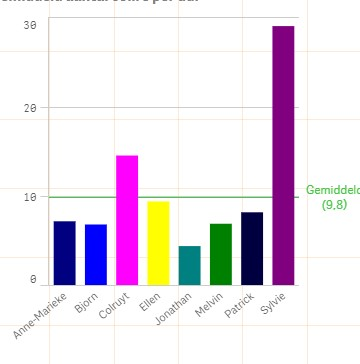# New to Qlik Sense

Discussion board where members can get started with Qlik Sense.

New Contributor III

## Static reference line

Dear community,

For a client of mine we hav this reference line:

=avg(total aggr(sum(\$(vColli))/\$(vDag),Datum, magazijnier))

Now if I Select something, the line adjusts.

But actually it should keep the total average line even when selecting a single person.

Here's a visual of the graph:Tags (1)
1 Solution

Accepted SolutionsMVP

## Re: Static reference line

Or this

=Avg(TOTAL {1} Aggr(Sum({1} \$(vColli))/\$(vDag), Datum, magazijnier))

8 RepliesPartner

## Re: Static reference line

maybe this:

=avg( {1} total aggr(sum(\$(vColli))/\$(vDag),Datum, magazijnier))

I hope it helps.MVP

## Re: Static reference line

Or this

=Avg(TOTAL {1} Aggr(Sum({1} \$(vColli))/\$(vDag), Datum, magazijnier))

New Contributor III

## Re: Static reference line

=avg(  total {1} aggr(sum({1}\$(vColli))/\$(vDag),Datum, magazijnier))

I think in Variable's expresssion  also, you need to modify:

\$(vColli)

\$(vDag)

New Contributor III

## Re: Static reference line

Gives back a completely different result :s

Highlighted
New Contributor III

## Re: Static reference line

modify how exactly?

thanks

New Contributor III

## Re: Static reference line

Amit is correct, what exactly vColli and vDag have as values?MVP

## Re: Static reference line

Would you be able to share a sample or an image to show what your issue is?

New Contributor III

## Re: Static reference line

Vcolli is a numeric value, if you have a crate with 10 articles in it, 1 article = 1colli

Vdag is a date (dag = day)